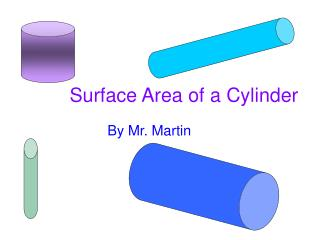DownloadDownload PresentationSurface Area of a Cylinder

# Surface Area of a Cylinder

Download Presentation## Surface Area of a Cylinder

- - - - - - - - - - - - - - - - - - - - - - - - - - - E N D - - - - - - - - - - - - - - - - - - - - - - - - - - -
##### Presentation Transcript

1. Surface Area of a Cylinder By Mr. Martin

2. Overview • Behold!A can with a: • Top (radius 3.75 cm) • Bottom (same as top), and • Label (11 cm high) Note: these are not the actual Measurements of the can.

3. The Top • Formula for Area Circle? • Area = Pi x r2 • Area= Pi x 3.752 • Area=3.14 (14.0625) • Area=44.15625 sq cm Radius (r)

4. The Bottom • Same as top • Area = Pi x r2 • Area= Pi x 3.752 • Area=3.14 (14.0625) • Area=44.15625 sq cm

5. Height The Label • What shape? • rectangle • Formula for area? • Height x width • Height? • 11cm Width • Width? • Pi x Diameter • Area = 11(3.14)(7.5)= 259.05 sq cm

6. Add Top, Bottom and Label • 44.15625 + 44.15625 + 259.05 = 347.3625 square cm Top Bottom

7. Now you try: Radius = 5 cm Height = 12 cm Note: These are not the actual dimensions of the can.